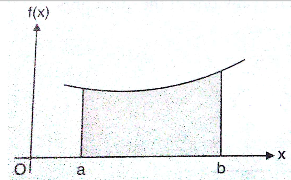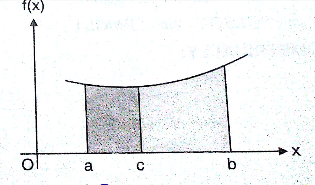Q

# Solve it, - Integral Calculus - JEE Main-2

The value of      denotes the greatest integer not exceeding is

• Option 1)

• Option 2)

• Option 3)

• Option 4)

119 Views

As learnt in concept

Fundamental Properties of Definite integration -

If the function is continuous in (a, b ) then integration of a function a to b will be same as the sum of integrals of the same function from a to c and c to b.

- whereinhas to be split into integral limits.

=

=

Terms start cancelling out,

We get,

=

Option 1)

This option is incorrect

Option 2)

This option is correct

Option 3)

This option is incorrect

Option 4)

This option is incorrect

Exams
Articles
Questions